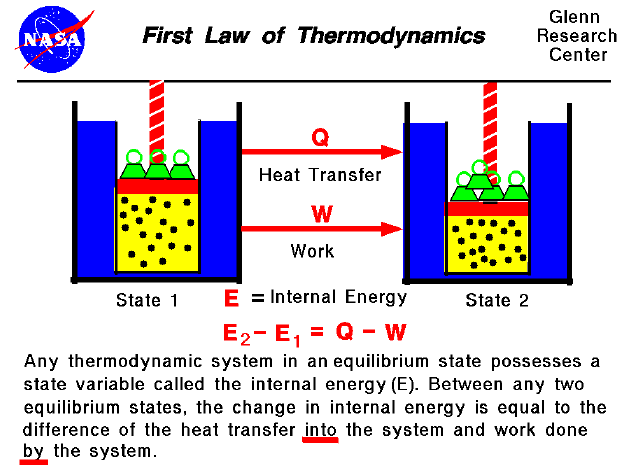Thermodynamics is a branch of physics which deals with the energy and work of a system. As mentioned on the gas properties slide, thermodynamics deals only with the large scale response of a system which we can observe and measure in experiments. In aerodynamics, the thermodynamics of a gas obviously plays an important role in the analysis of propulsion systems but also in the understanding of high speed flows.

In our observations of the work done on (or by) a gas, we have found that the amount of work depends not only on the initial and final states of the gas but also on the process (or path) which produces the final state. Similarly the amount of heat transferred into (or from) a gas also depends on the initial and final states and the process which produces the final state. Many observations of real gases have shown that the difference of the heat flow into the gas and the work done by the gas depends only on the initial and final states of the gas and does not depend on the process or path which produces the final state. This suggests the existence of an additional variable, called the internal energy of the gas, which depends only on the state of the gas and not on any process. The internal energy is a state variable, just like the temperature or the pressure. The first law of thermodynamics defines the internal energy (E) as equal to the difference of the heat transfer (Q) into a system and the work (W) done by the system. (E2 - E1 = Q - W) We have emphasized the words "into" and "by" in the definition. Heat removed from a system would be assigned a negative sign in the equation. Similarly work done on the system is assigned a negative sign.

The internal energy is just a form of energy like the potential energy of an object at some height above the earth, or the kinetic energy of an object in motion. In the same way that potential energy can be converted to kinetic energy while conserving the total energy of the system, the internal energy of a thermodynamic system can be converted to either kinetic or potential energy. Like potential energy, the internal energy can be stored in the system. Notice, however, that heat and work can not be stored or conserved independently since they depend on the process. The first law of thermodynamics allows for many possible states of a system to exist, but only certain states are found to exist in nature. The second law of thermodynamics helps to explain this observation.

If a system is fully insulated from the outside environment, it is possible to have a change of state in which no heat is transferred into the system. Scientists refer to a process which does not involve heat transfer as an adiabatic process. The implementation of the first law of thermodynamics for gases introduces another useful state variable called the enthalpy which is described on a separate page.

Guided Tours